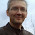## Saturday, October 3, 2020

### TI-84 Plus CE and HP 41C: Method of Successive Substitutions

TI-84 Plus CE and HP 41C:  Method of Successive Substitutions

Repeating the Calculation Again and Again

With an aid of a scientific calculator, we can solve certain problems of the form:

f(x) = x.

Examples include:

tan cos sin x = x

e^-x = x

atan √x = x

sin cos x = x

(acos x) ^ (1/3) = x

In any case with trigonometric functions, the angle mode will need to be in radians.  You will also need a good guess to get to a solution and to know that at some real number x, f(x) and x intersect.

Take the equation ln(3*x) = x with initial guess x0 = 1.512

Depending on the operating of the scientific calculator the keystrokes would be:

AOS:

1.512 [ = ]

Loop:  [ × ] 3 [ = ] [ ln ]

RPN:

1.512 [ENTER]

Loop:  3 [ × ] [ ln ]

ALG:

1.512 [ENTER/=]

Loop:  ln( 3 * Ans) [ ENTER/= ]

Repeat the loop as many times as you like and hope you start seeing the answers converge.   After repeating the loop over and over and over again, at six decimal answers, the readout will be about 1.512135.

An approximate answer to ln(3x) = x, x ≈ 1.512134552

If your calculator has a solve function, you can check the answer, but this method can be useful if your calculator does not have a solve function.

The program SUCCESS illustrates this method.

TI-84 Plus CE Program (TI-83 Family) Program:  SUCCESS

285 bytes

"2020-09-08 EWS"

Disp "SUCCESIVE SUBSTITUTION", "F(X)=X"

Disp "USE QUOTES FOR F(X)"

Input "F(X)? ", Y1

Input "GUESS? ", G

Input "PRECISION? ", P

0 → I

1 → T

Repeat T<10^-P

Y1 → Z

abs(Z - X) → T

Z → X

I + 1 → I

If I > 200

Then

Disp "ITERATIONS EXCEEDED", "NO SOLUTION FOUND"

Stop

End

End

ClrHome

Disp "SOLUTION=",  round(X,P), "ITERATIONS=", I, "ABS DIFF", T

HP 41C/DM41 Program: SUCCESS

This program calls on the subroutine, FX.   FX is where you enter f(x).  End FX with the RTN command.

01 LBL^T SUCCESS

02 ^T F<X>=X

03 AVIEW

04 PSE

05 ^T GUESS?

06 PROMPT

07 STO 00

08 ^T PRECISION?

09 PROMPT

10 STO 02

11 0

12 STO 03

13 1

14 STO 04

15 LBL 01

16 RCL 00

17 XEQ ^FX

18 STO 01

19 RCL 00

20 -

21 ABS

22 STO 04

23 RCL 01

24 STO 00

25 1

26 ST+ 03

27 200

28 RCL 03

29 X>Y?

30 GTO 02

31 RCL 02

32 CHS

33 10↑X

34 RCL 04

35 X>Y?

36 GTO 01

37 ^T SOL=

38 ARCL 01

39 AVIEW

40 STOP

41 ^T ITER=

42 ARCL 03

43 AVIEW

44 STOP

45 ^T DIFF=

46 ARCL 04

47 AVIEW

48 STOP

49 GTO 04

50 LBL 02

51 ^T NO SOL FOUND

52 AVIEW

53 STOP

54 LBL 04

55 END

Examples for FX:

f(x) = sin cos x.

Program:

LBL ^FX

COS

SIN

RTN

f(x) = e^-x

Program:

LBL ^FX

CHS

E↑X

RTN

Be aware, some equations cannot be solved in this manner, such as x = π / sin x and x = ln(1 / x^4).

Cheung, Y.L. "Using Scientific Calculators to Demonstrate the Method of Successive Substitutions"  The Mathematics Teacher.  National Council of Teachers of Mathematics.  January 1986, Vol. 79 No. 1 pp. 15-17  http://www.jstor.com/stable/27964746

Eddie

All original content copyright, © 2011-2020.  Edward Shore.   Unauthorized use and/or unauthorized distribution for commercial purposes without express and written permission from the author is strictly prohibited.  This blog entry may be distributed for noncommercial purposes, provided that full credit is given to the author.

#### 1 comment:

1.Hi, Eddie! This is named as "Fixed point iteration" ( https://en.wikipedia.org/wiki/Fixed-point_iteration ) and yes, this is a well known method.

### HP 42S/DM42: Height of a Fire, Time to Clear a Corridor

HP 42S/DM42:  Height of a Fire, Time to Clear a Corridor HP 42S/DM42/Free42 Program:  FIREHGT The program FHGT approximates the height of a...